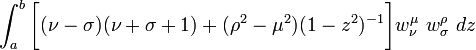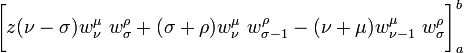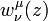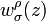User:Tohline/Math/EQ Toroidal05$~ \int_a^b\biggl[(\nu - \sigma)(\nu + \sigma + 1) + (\rho^2 - \mu^2)(1 - z^2)^{-1} \biggr] w_\nu^\mu ~w_\sigma^\rho ~dz$$~=$$~ \biggl[ z(\nu-\sigma) w_\nu^\mu ~w_\sigma^\rho + (\sigma+\rho) w_\nu^\mu ~ w_{\sigma-1}^\rho - (\nu + \mu) w_{\nu - 1}^\mu ~w_\sigma^\rho \biggr]_a^b$ A. Erdélyi (1953):  Volume I, §3.12, p. 169, eq. (1)
 where,$~w_\nu^\mu(z)$ and$~w_\sigma^\rho(z)$ denote any solutions of Legendre's differential equation